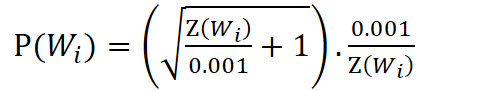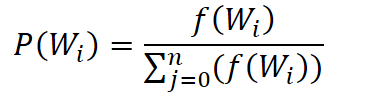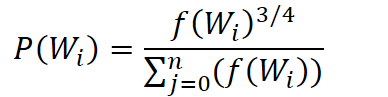TowardsMachineLearning# Introduction

Recall that our neural network had two weight matrices–a hidden layer and output layer. Both of these layers would have a weight matrix with 300 x 10,000 = 3 million weights each. These results in a skip-gram neural network that contains a huge number of weights.

Running gradient descent on a neural network that large is going to be slow. And to make matters worse, you need a huge amount of training data in order to tune that many weights and avoid over-fitting. Millions of weights times billions of training samples means that training this model is going to be a beast.

The authors of word2vec applied a few sampling techniques to their models which both reduce the compute requirements dramatically and improved the quality of the word vectors learned. In summary:

• They subsample frequent words to decrease the number of training examples.
• The context window is shrunk by random amounts to give greater weight to closer context words.
• They modified the optimization objective with a technique they called “Negative Sampling“, which causes each training sample to update only a small percentage of the model’s weights.

## 1. Subsampling Frequent Words-

In our below example ,I’ve used a small window size of 2 just for the example. The word highlighted in brown color is the input word.

There are two “problems” with common words like “the”:

•  When looking at word pairs, (“the“, “and”) doesn’t tell us much about the meaning of “Modi”. “the” appears in the context of pretty much every word.
• We will have many more samples of (“the“, …) than we need to learn a good vector for “the”.

Since such frequent words often provide little information .So the words with frequency above a certain threshold (e.g ‘a’, ‘an’ and ‘that’) may be subsampled to increase training speed and performance. Also, common word pairs or phrases may be treated as single “words” to increase training speed.

The word2vec implements an equation for calculating a probability with which to keep a given word in the vocabulary. In this equation, 𝑤𝑖 is the word, and 𝑧(𝑤𝑖) is the fraction of the total words in the corpus that are that word. For example, if the word “peanut” occurs 1,000 times in a 1 billion word corpus, then z(‘peanut’) = 1E-6. There is also a parameter in the code named ‘sample’ which controls how much subsampling occurs, and the default value is 0.001. Smaller values of ‘sample’ mean words are less likely to be kept.

P({{W}_{i}}) is the probability of keeping the word:Word2vec effectively weights context words differently based on their position within the context window. Or, more specifically, context words closer to the center word are given more weight than words farther out. This is not implemented in the math or architecture of the neural network, though! Instead, this weighting is achieved by randomly shrinking the size of the context window.

In the word2vec C code, each time it moves the context window to the next word in a sentence, it
picks a random window size in the range [1,window_size]. This means that if you
train word2vec with, for example, a window size of 4, then the actual window size
will vary uniformly between 1 and 4. The following illustration shows how this
might play out with a window size of 4.Since all window sizes in the range are equally probable, each context position has a proportional probability of being included. Here are some examples for different maximum window sizes; the percentage in the box is the percentage of training samples that will include the word at that context position.## 3. Negative Sampling-

Training a neural network means taking a training example and adjusting all of the neuron weights slightly so that it predicts that training sample more accurately. In other words, each training sample will tweak all of the weights in the neural network.

As we discussed above, the size of our word vocabulary means that our skip-gram neural network has a tremendous number of weights, all of which would be updated slightly by every one of our billions of training samples.

Negative sampling addresses this by having each training sample only modify a small percentage of the weights, rather than all of them. Here’s how it works-

When training the network on the word pair (“PM”, “Modi”), recall that the “label” or “correct output” of the network is a one-hot vector. That is, for the output neuron corresponding to “Modi” to output a 1, and for all of the other thousands of output neurons to output a 0. With negative sampling, we are instead going to randomly select just a small number of “negative” words (let’s say 5) to update the weights for. (In this context, a “negative” word is one for which we want the network to output a 0 for). We will also still update the weights for our “positive” word (which is the word “quick” in our current example).

Recall that the output layer of our model has a weight matrix that’s 300 x 10,000. So we will just be updating the weights for our positive word (“Modi”), plus the weights for 5 other words that we want to output 0. That’s a total of 6 output neurons, and 1,800 weight values total. That’s only 0.06% of the 3M weights in the output layer!

In the hidden layer, only the weights for the input word are updated (this is true whether
you’re using Negative Sampling or not).

## Selecting Negative Samples-

The “negative samples” (that is, the 5 output words that we’ll train to output 0) are selected using a “unigram distribution”, where more frequent words are more likely to be selected as negative samples. For instance, suppose you had your entire training corpus as a list of words, and you chose your 5 negative samples by picking randomly from the list. In this case, the probability for picking the word “couch” would be equal to the number of times “couch” appears in the corpus, divided the total number of words in the corpus. This is expressed by the following equation:The authors state in their paper that they tried a number of variations on this equation, and the one which performed best was to raise the word counts to the ¾ power.If you play with some sample values, you’ll find that, compared to the simpler equation, this one has the tendency to increase the probability for less frequent words and decrease the probability for more frequent words.

The way this word selection is implemented in the C code is interesting. They have a large array with 100M elements (which they refer to as the unigram table). They fill this table with the index of each word in the vocabulary multiple times, and the number of times a word’s index appears in the table is given by 𝑃(𝑤𝑖) * table_size. Then, to actually select a negative sample, you just generate a random integer between 0 and 100M, and use the word at that index in the table. Since the higher probability words occur more times in the table, you’re more likely to pick those.

## End Notes:-

In this article, I’ve tried to walk you through the possible challenges in Word2vec Model, and different solutions to overcome them.

You can read other articles related to Word2vec here-

## Article Credit:-

Name:  Praveen Kumar Anwla

Founder – Towards Machine Learning